×#### Thank you for registering.

One of our academic counsellors will contact you within 1 working day.

Click to Chat

1800-1023-196

+91-120-4616500

CART 0

• 0

MY CART (5)

Use Coupon: CART20 and get 20% off on all online Study Material

ITEM
DETAILS
MRP
DISCOUNT
FINAL PRICE
Total Price: Rs.

There are no items in this cart.
Continue Shopping• Complete JEE Main/Advanced Course and Test Series
• OFFERED PRICE: Rs. 15,900
• View Details

```Chapter 18: Basic Geometrical Tools Exercise 18.1

Question: 1

Construct the following angles using set- squares:

(i) 45°

(ii) 90°

(iii) 60°

(iv) 105°

(v) 75°

(vi) 150°

Solution: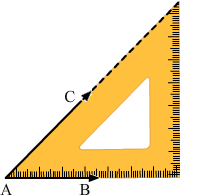(i) 45°

Place 45° set- square.

Draw two rays AB and AC along the edges from the vertex from the vertex of 45o angle of the set- square.

The angle so formed is a 45° angle.

∠BAC = 45°

(ii) 90°

Place = 90° set –square as shown in the figure.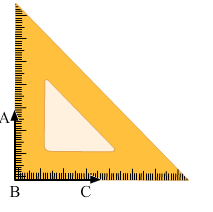Draw two rays BC and BA along the edges from the vertex of 90° angle.

The angle so formed is 90° angle.

∠ABC = 90°

(iii) 60°

Place 30° set –square as shown in the figure.

Draw the rays BA and BC along the edges from the vertex of 60°

The angle so formed is 60°

∠ABC = 60°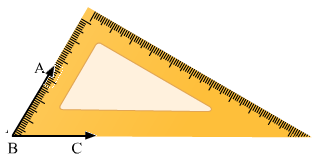(iv) 105°

Place 30° set –square and make an angle 60° by drawing the rays BA and BC as shown in figure.Now place the vertex of 45°of the set –square on the ray BA as shown in figure and draw the ray BD.

The angle so formed is 105°

Therefore, ∠DBC = 105°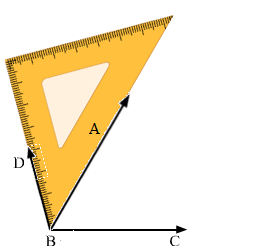(v) 75o

Place 45° set –square and make an angle of 45° by drawing the rays BD and BC as shown in the figure.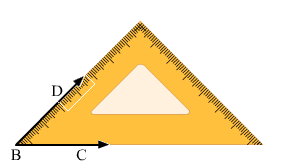Now place the vertex of 30° of the set- square on the ray BD as shown in the figure and draw the ray BA.

The angle so formed is 75°.

Therefore, ∠ABC = 75°(Line BD is hidden)

(vi) 150°

Place the vertex of 45° of the set – square and make angle of 90o by drawing the rays BD and BC as shown in the figure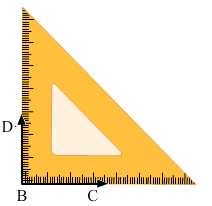Now, place the vertex of 30°of the set –square on the ray BS as shown in the figure and draw the ray BA

The angle so formed is 150°.

Therefore, ∠ABC = 150°Question: 2

Given a line BC and a point A on it, construct a ray AD using set – squares so that ∠DAC is

(i) 30°

(ii) 150°

Solution:

(i) Draw a line BC and take a point A on it. Place 30° set –square on the line BC such that its vertex of 30° angle lies on point A and one edge coincides with the ray AB as shown in figure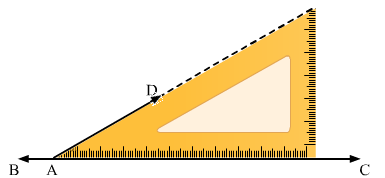Thus ∠DAC is the required angle of 30°

(ii) Draw a line BC and take a point A on it. Place 30° set –square on the line BC such that its vertex of 30° angle lies on point A and one edge coincides with the ray AB as shown in the figure.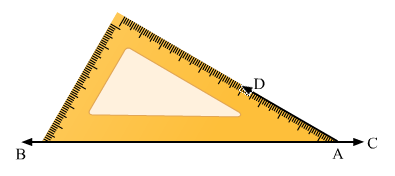Therefore, ∠DAB = 30°

We know that angle on one side of the straight line will always add to 180o

Therefore, ∠DAB + ∠DAC = 180°

Therefore, ∠DAC = 150°
```### Course Features

• 728 Video Lectures
• Revision Notes
• Previous Year Papers
• Mind Map
• Study Planner
• NCERT Solutions
• Discussion Forum
• Test paper with Video Solution